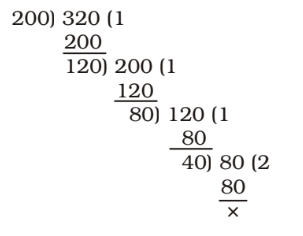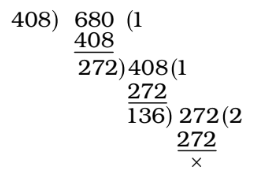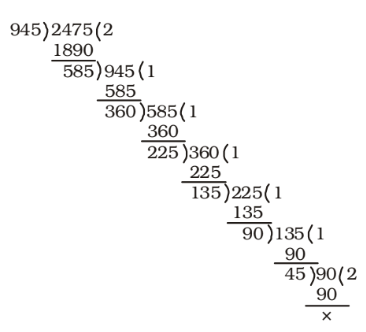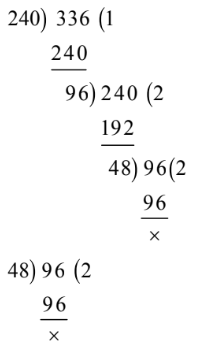## LCM and HCF

#### LCM and HCF

1. Find the greatest number which will exactly divide 200 and 320.

1. Firstly , We find HCF of 200 and 320Required number = HCF of 200 and 320

##### Correct Option: D

Required number = HCF of 200 and 320
Firstly , We find HCF of 200 and 320Required number = HCF of 200 and 320 = 40

1. The greatest number that divides 411, 684, 821 and leaves 3, 4 and 5 as remainders, respectively, is

1. We know that the largest number which when divide the numbers a, b and c give remainders as p, q, r respectively is given by H.C.F. of (a – p), (b – q) and (c – r).
Required number = HCF of 411 – 3 = 408; 684 – 4 = 680 and 821 – 5 = 816
HCF of 408 and 816 = 408
HCF of 408 and 680∴ Required number = HCF of 408 and 680

##### Correct Option: C

We know that the largest number which when divide the numbers a, b and c give remainders as p, q, r respectively is given by H.C.F. of (a – p), (b – q) and (c – r).
Required number = HCF of 411 – 3 = 408; 684 – 4 = 680 and 821 – 5 = 816
HCF of 408 and 816 = 408
HCF of 408 and 680∴ Required number = HCF of 408 and 680
Hence , Required number = 136

1. A milk vendor has 21 litres of cow milk, 42 litres of toned milk and 63 litres of double toned milk. If he wants to pack them in cans so that each can contains same litres of milk and does not want to mix any two kinds of milk in a can, then the least number of cans required is

1. Maximum quantity in each can = HCF of 21, 42 and 63 litres = 21 litres

 Required least number of cans = 21 + 42 + 63 21 21 21

##### Correct Option: B

Maximum quantity in each can = HCF of 21, 42 and 63 litres = 21 litres

 Required least number of cans = 21 + 42 + 63 21 21 21

Required least number of cans = 1 + 2 + 3 = 6

1. A farmer has 945 cows and 2475 sheep. He farms them into flocks, keeping cows and sheep separate and having the same number of animals in each flock. If these flocks are as large as possible, then the maximum number of animals in each flock and total number of flocks required for the purpose are respectively

1. First of all we find the HCF of 945 and 2475. HCF = 45
Illustration :∴ Maximum number of animals in each flock = 45

 Required total number of flocks = 945 + 2475 45 45

##### Correct Option: C

First of all we find the HCF of 945 and 2475. HCF = 45
Illustration :∴ Maximum number of animals in each flock = 45

 Required total number of flocks = 945 + 2475 = 21 + 55 = 76 45 45

1. Three sets of English, Mathematics and Science books containing 336, 240, 96 books respectively have to be stacked in such a way that all the books are stored subject-wise and the height of each stack is the same. Total number of stacks will be

1. Number of books in each stack = HCF of 336, 240, 96 = 48∴ Total number of stacks = 336 + 240 + 96 48 48 48

##### Correct Option: A

Number of books in each stack = HCF of 336, 240, 96 = 48∴ Total number of stacks = 336 + 240 + 96 48 48 48

Hence , Total number of stacks = 7 + 5 + 2 = 14